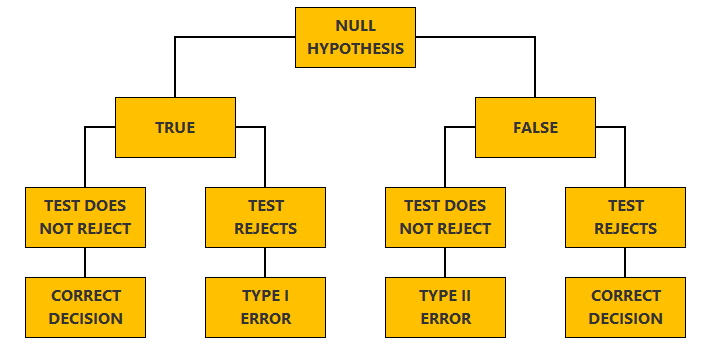StatLect

Type II error

In hypothesis testing, a Type II error occurs when the null hypothesis is not rejected even though it is false.Synonyms

Type II errors are also referred to as errors of the second kind.

Explanation

When performing a test of hypothesis, a decision is made as to whether the null hypothesis should be rejected or not. This choice is made on the basis of a test statistic. When the value taken by the statistic belongs to a critical region that has been specified in advance, the null hypothesis is rejected. Otherwise, the null is not rejected. This decision process is prone to the following kind of error: do not reject the null hypothesis when the null hypothesis is indeed false. Errors of this kind are dubbed Type II errors, in order to distinguish them from Type I errors, which occur when the null hypothesis is rejected despite being true.

The next tree plot provides a graphical illustration of the two types of error.Probability of Type II errors

Earlier we have mentioned that the outcome of a test of hypothesis depends on the value assumed by a test statistic. In turn, this value depends on the data used to compute the statistic. But the data is random. Thus, before the data is observed, the test statistic can be regarded as a random variable. As a consequence, the probability that a Type II error occurs is equal to the probability that the realization of a random variable (the test statistic) will not belong to the critical region.

In several important cases, the probability of committing Type II errors can be exactly quantified through a function called power function.

Example

Suppose you make an hypothesis about the variance of a probability distribution. In particular, your null hypothesis is that the variance of the distribution should be equal to 1. After formulating your hypothesis, you collect a sample of observations from the distribution, and, based on the sample variance, you do not reject the null hypothesis that the variance of the distribution is equal to 1. If instead the true (and unknown) variance of the distribution is not equal to 1 (for example, because it is equal to 2), then you are committing a Type II error.

A thorough discussion of Type II errors committed while performing tests of hypotheses about the variance can be found in the lecture entitled Hypothesis tests about the variance.

More details

Go to the lecture entitled Hypothesis testing for more details about Type II errors.